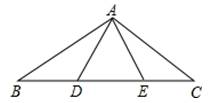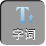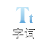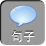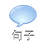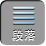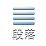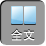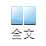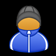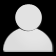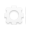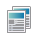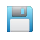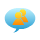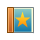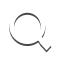-AA+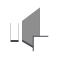1、（ 2009•攀枝花）如图所示，在等边△ ABC中，点 DE分别在边 BCAB上，且 BD= AEADCE交于点 F，则∠ DFC的度数为（）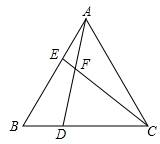∵△ ABC为等边三角形

∴∠ BAC=∠ B=∠ BCA= 60°

AB= BC= AC

BD= AE，∠ ABD=∠ CAEAB= AC

∴△ ABD≌△ CAE

∴∠ BAD=∠ ACE

∴∠ ACE+∠ DAC= 60

∵∠ ACE+∠ DAC+∠ AFC= 180°

∴∠ AFC= 120

∵∠ AFC+∠ DFC= 180

∴∠ DFC= 60°．

2、（ 2006•天津）如图， ACB三点在同一条直线上，△ DAC和△ EBC都是等边三角形， AEBD分别与 CDCE交于点 MN，有如下结论：①△ ACE≌△ DCB；② CM= CN；③ AC= DN．其中正确结论是（）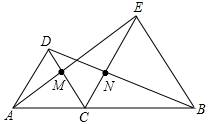∵⊿ ADC和⊿ BCE是等边三角形

AC= CDCE= BC，∠ ACD=∠ ECB= 60°

∴∠ ACD+∠ DCE=∠ ECB+∠ DCE

∴∠ ACE=∠ DCB

∴△ ACE≌△ DCBSAS）（①正确）

∴∠ AEC=∠ DBC

∵∠ DCE+∠ ACD+∠ ECB= 180°，∠ ACD=∠ ECB= 60°

∴∠ DCE=∠ ECB= 60°

CE= BC，∠ DCE=∠ ECB= 60°，∠ AEC=∠ DBC

∴△ EMC≌△ BNCASA

CM= CN（②正确）

AC= DC在△ DNC中， DC所对的角为∠ DNC=∠ NCB+∠ NBC= 60°+∠ NBC60°，而 DN所对的角为 60°，根据三角形中等边对等角、大边对大角，小边对小角的规律，则 DCDN，即是 ACDN，所以③错误。

3、（ 2005•郴州）附加题：下图是由九个等边三角形组成的一个六边形，当最小的等边三角形边长为 2 cm时，这个六边形的周长为（） cm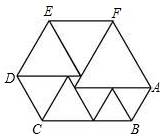∴六边形周长是 2 x+ 2x+ 2）+ 2x+ 2× 2）+（ x+ 3× 2）= 7 x+ 18，而最大的三角形的边长 AF等于 ABANOAHDIGITAL 10倍，所以可以求出 x，则可求得周长．

∴等边三角形的边长依次为 xx+ x+ 2x+ 2x+ 2× 2x+ 2× 2x+ 3× 2

∴六边形周长是 2 x+ 2x+ 2）+ 2x+ 2× 2）+（ x+ 3× 2）= 7 x+ 18

AF= 2 AB，即 x+ 6= 2 x

x= 6 cm

∴周长为 7 x+ 18= 60 cm